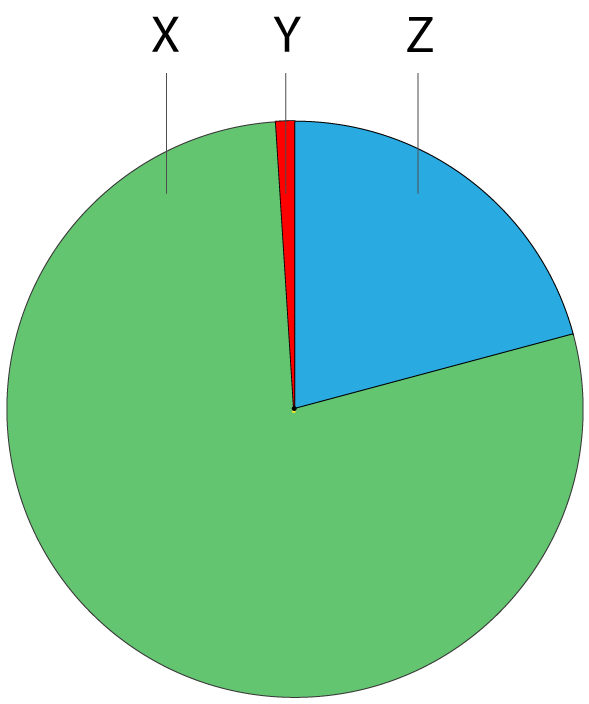1. The pie chart shows the approximate percentage of the three most abundant gases in dry air in today’s atmosphere.

The gases represented by X, Y and Z are:X Y Z A oxygen carbon dioxide nitrogen B nitrogen Carbon dioxide oxygen C oxygen argon nitrogen D nitrogen argon oxygen
2. The test for oxygen gas is …
• A.   relights a glowing splint
• B.   ignites with a pop
• C.   turns limewater milky
• D.   a lit splint continues to burn

Q3-5:
A student set up the following apparatus to determine the percentage of oxygen in polluted air. He left the apparatus set up until the water level stopped rising and remained constant.

He obtained the following results:

• Initial volume of gas in the tube = 150 cm3
• Final volume of gas in the tube = 122 cm33. The percentage of oxygen, to the nearest whole number, in the polluted air is..
• A.   81%
• B.   28%
• C.   21%
• D.   19%
4. What would be the effect, if any, on the calculated percentage of oxygen in the air if the student left the experiment set up for longer?
5. What would be the effect, if any, on the calculated percentage of oxygen in the air if the student did not use excess iron wool?

6. What process produced the gases in the Earth’s early atmosphere?

• A.   Photosynthesis by trees and vegetation
• B.   Volcanic activity
• C.   Respiration of pre-historic creatures
• D.   Decaying vegetation

7. The composition of the Earth’s early atmosphere is uncertain but has been determined by analyzing evidence.

Which of the following was the Earth’s early atmosphere NOT thought to contain?

• A.   a large amount of oxygen
• B.   a large amount of carbon dioxide
• C.   water vapour
• D.   small amounts of gases such as methane and ammonia
Q8-9:
A sample of clean, dry air is passed over hot copper until all the oxygen in the air has reacted with the copper forming copper(II)oxide.8. What is the correct equation for the reaction occurring?
• A. 2Cu(s) +   O2(g)  2CuO(s)
• B.  Cu(s) +   O2(g)  CuO2(s)
• C. 4Cu(s) +   O2(g)  2Cu2O(s)
• D. 2Cu(s) +   O2(g)  Cu2O2(s)
9. If the volume of air decreased by 20 cm3 what was the starting volume of the air?
• A.   40 cm3
• B.   60 cm3
• C.   100 cm3
• D.   200 cm3
10. Which of the following statements about the changes in the early atmosphere is NOT true?
• A.   Water vapour condensed forming oceans.
• B.   Photosynthesis of primitive plants caused oxygen levels to increase
• C.   Carbon dioxide dissolved in the oceans
• D.   Respiration of primitive animals caused oxygen levels to fall